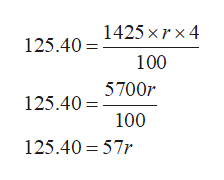Use the simple interest formula to determine the missing value. p =\$1425,r= ?t= 4 yearsi= \$125.40 ​

Question
Use the simple interest formula to determine the missing value.

p =\$1425,
r= ?
t= 4 years
i= \$125.40

Step 1

Given information :

P =1425;

t=4 years

i=125.40

here, let rate, r %

Now, use the following formula to find the required value:

Step 2

Now, plug all the provided values:help_outlineImage Transcriptionclose1425 xr x 4 125,401425 x r x 4 100 125.40700r 100 125.40 57r fullscreen
Step 3

Now, further simplify by divi...

Want to see the full answer?

See Solution

Want to see this answer and more?

Our solutions are written by experts, many with advanced degrees, and available 24/7

See Solution
Tagged in

Math